# Crystal UnitsGlossary

Basic Knowledge of Crystal Units

No. Item Title Contents
1 Technical terms Resonance frequency

In the resonance characteristic of the crystal unit, resonance frequency is lower frequency one in which two points impedance becomes only resistive.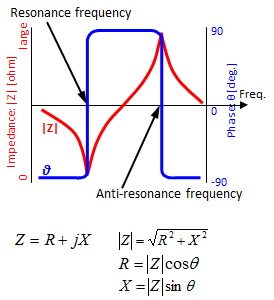The frequencies that impedance Z becomes only a resistance element exist at two points. At these points, the phase is 0.
One with low frequency is called a resonance frequency among them. The other is called an anti-resonance frequency.

2 Technical terms Equivalent circuit

The figure below is showing the resonance characteristic of the crystal unit by resistance, inductance, and capacitance. R1 in the equivalent circuit is called equivalent series resistance and important characteristics of the crystal.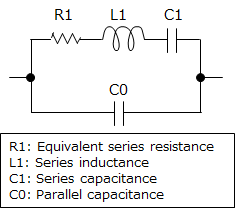3 Technical terms Equivalent series resistance(R1) The resistance in the series arm of the equivalent circuit of the crystal.
4 Technical terms Load capacitance (Cs)

It is a capacitance gives load resonance frequency with the crystal.
In actual oscillation circuit, it is virtual capacitance connected to crystal given by external load capacitors, stray of IC, PCB and so on.
It is able to calculate by formula blow.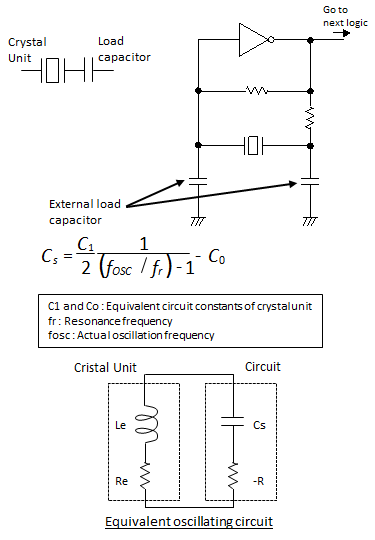5 Technical terms Load resonance frequency (fL)

The load resonance frequency is resonance frequency with load capacitor series in the crystal unit, and this frequency is higher than resonance frequency.
Frequency difference will exist between actual and nominal oscillation frequency due to capacitance difference between actual and specified value in crystal unit's specification.
It is able to calculate by formula blow.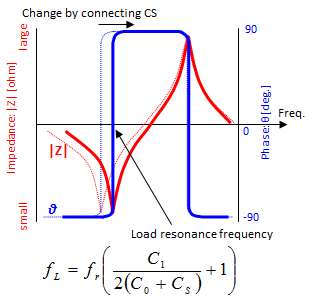6 Technical terms Pulling sensitivity

The above chart shows load resonance frequency (fL) shift by load capacitance change.
The slop of each point in this chart becomes pulling sensitivity.
Please refer the following chart. When the load capacitance is 6pF, the pulling sensitivity is -17ppm/pF. (frequency will shift 17ppm by 1pF change of load capacitance.)
It is able to calculate by formula below.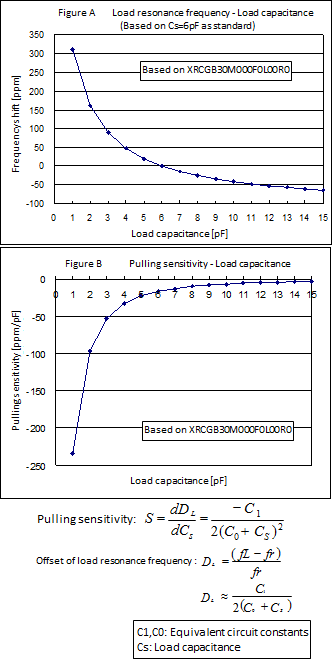It is resonance characteristic of the crystal plotted on admittance plane (conductance-susceptance) coordinates. It is called admittance circle because it draws circle.
If frequency is lower than resonant frequency, admittance is in near the origin.
Adimittance draws clockwise circle by frequency rising.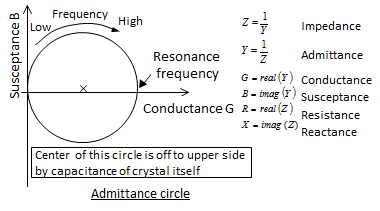8 Technical terms Oscillation margin / Negative resistance analysis It is a margin to the oscillation stop and the most important item in the oscillation circuit.
Oscillation margin depends on parts (crystal unit, MCU, capacitor, and resistor) that compose the oscillation circuit.
Murata recommends to keep oscillation margin 5 times or more.
Please refer "explanation of oscillation margin" in detail.
9 Technical terms Negative resistance (-R)

Negative resistance is the capability of the signal amplification of the oscillation circuit indicated by resistance.
It has negative value because it works oppositely to resistance.
Small absolute value of negative resistance shows low amplification capability of oscillation circuit.
Negative resistance in the oscillation circuit is depending on CMOS inverter characteristic,the feedback resistance, damping resistance and the external load capacitance.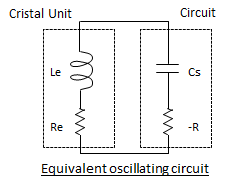10 Technical terms Drive level The drive level indicates the power consumption of the crystal unit in the oscillation circuit.
It depends not only on the equivalent series resistance of the crystal but also parts (MCU, capacitance, and resistance) that compose the oscillation circuit.
Unusual characteristics might appear in frequency-temperature characteristics when the drive level is excessive. It is better to check drive level when you design oscillation circuit.
11 Technical terms C-MOS inverter

C-MOS is complementary MOS and composed p and n type MOSFET connected complementally.
It works as inverter (logical invertering circuit.NOT) in the shape of the figure below.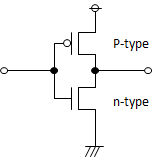12 Technical terms Oscillation circuit

In the kind of amplifying circuit with C-MOS inverter or transistor, it is called "oscillation circuit" that output is connected to input to make feedback to continue amplification.
Only the signal at the resonance frequency is selected and amplified by feeding back through crystal unit.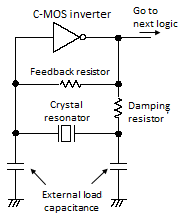13 Technical terms Circuit matching Oscillation characteristic changes by combination of parts (C-MOS inverter, crystal unit, resistance, and external load capacitor) that compose the circuit.
Therefore, it is necessary to apply suitable combination of capacitance to obtain robust oscillation. This check and adjustment is called circuit matching.
14 Technical terms Nominal frequency The nominal frequency indicates the frequency that the crystal unit manufacturer specifies for the crystal.
It is necessary to understand that actual oscillation might shift from nominal frequency by difference of MCU,
15 Technical terms Frequency tolerance It is frequency range that the maximum permissible deviation of the oscillating frequency in the operation conditions. It is generally shown by ppm based on the nominal frequency.
16 Oscillation circuit parts Feedback resistor The feedback resister is connected to the C-MOS inverter in parallel in the oscillation circuit. It might be integrated into MCU.
It has role to balance DC voltage between the I/O of the inverter, and the inverter will work as an amplifier.
When the feedback resister is not integrated into MCU, It is better to apply 1Mohm as external to feedback resistor.
17 Oscillation circuit parts Damping resistor The damping resistor is applied at output side of the C-MOS inverter in the oscillation circuit. It has role to attenute oscillation amplitude for reducing drive level. On the other hands, it is necessary to pay attention for oscillation margin because excessive damping resistance may cause oscillation stop.
Damping resistance is generally used in the range from 0 to 2kΩ, and it depends on the characteristic of MCU.
18 Oscillation circuit parts External load capacitor The external load capacitors are applied input side and the output side of the inverter to ground in the oscillation circuit.
They are important parts that influence negative resistance and the oscillation frequency directly.
These capacitors are called "Load capacitor" in CERALOCK. On the other hand, in the crystal unit, it is called "External load capacitor" to distinguish from load capacitor "Cs".
Two same capacitors are usually used as external load capacitor.
It would be suitable that 5 to 10pF as external load capacitance, and it depends on the characteristic of MCU and the parasitic capacitance of the mounting substrate.
19 Measuring method Oscillation margin Refer to another material
20 Measuring method Oscillation margin (Simple method) Refer to another material
21 Measuring method Drive level Refer to another material
22 Measuring method
Oscillating frequency Refer to another material
23 Measuring method
Oscillating amplitude Refer to another material